book

flag
mode_edit

## 红黑树

### 性质

1. 节点是红色或黑色；
2. 从每个叶子到根的所有路径上不能有两个连续的红色节点；
3. 从任一节点到其每个叶子的所有简单路径都包含相同数目的黑色节点。（黑高平衡）

## 左偏红黑树（Left Leaning Red Black Tree）

• 要么全是黑色；
• 要么左儿子是红色，右儿子是黑色。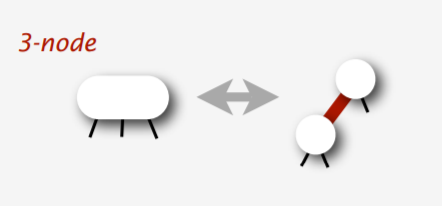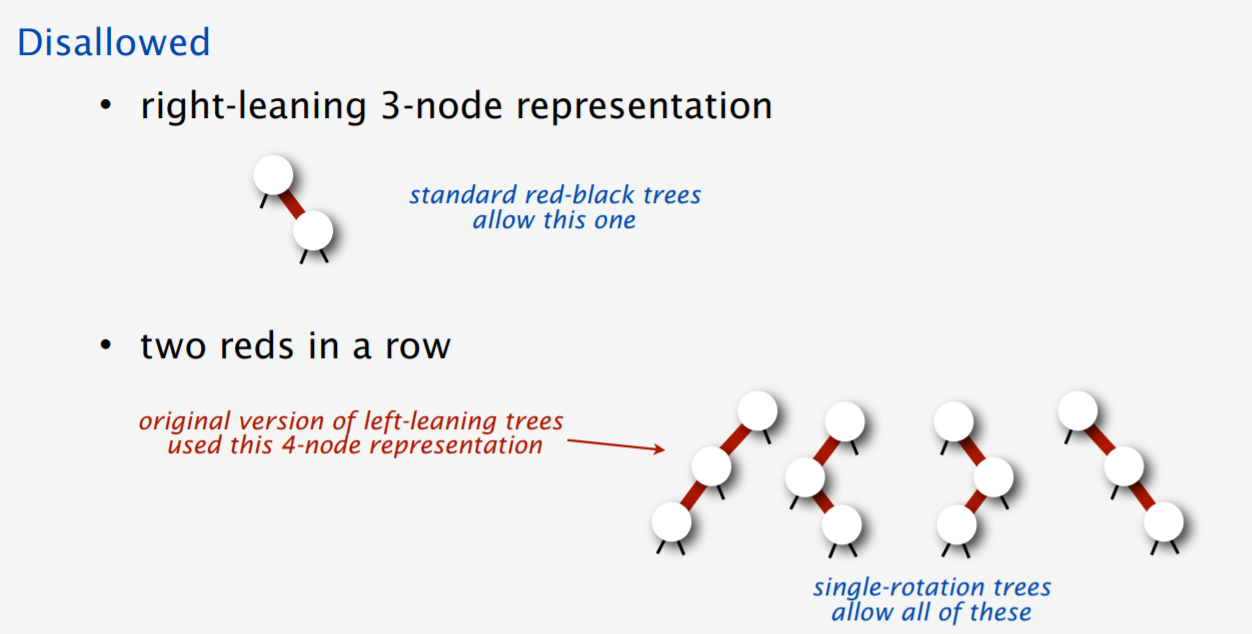### 插入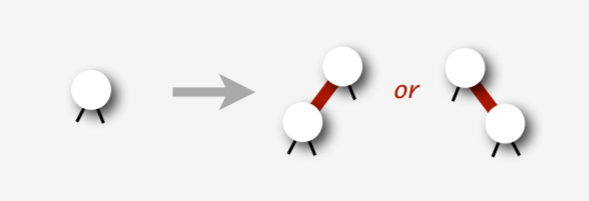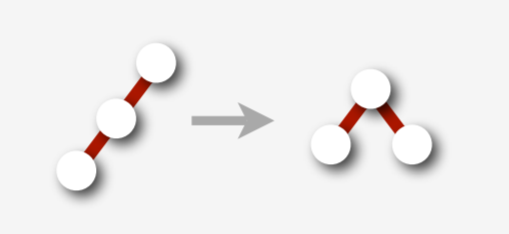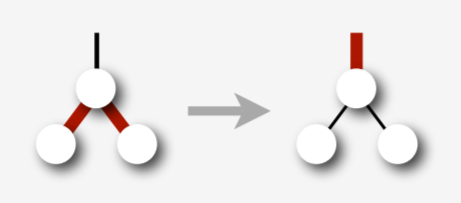template<class Key, class Compare>
typename Set<Key, Compare>::Node *
Set<Key, Compare>::fix_up(Set::Node *root) const {
if (is_red(root->rc) && !is_red(root->lc)) // fix right leaned red link
root = rotate_left(root);
if (is_red(root->lc) && is_red(root->lc->lc)) // fix doubly linked left leaned red link
// if (root->lc == nullptr), then the second expr won't be evaluated
root = rotate_right(root);
if (is_red(root->lc) && is_red(root->rc))
// break up 4 node
color_flip(root);
root->size = size(root->lc) + size(root->rc) + 1;
return root;
}

template<class Key, class Compare>
typename Set<Key, Compare>::Node *
Set<Key, Compare>::insert(Set::Node *root, const Key &key) const {
if (root == nullptr)
return new Node(key, kRed, 1);
if (root->key == key);
else if (cmp_(key, root->key)) // if (key < root->key)
root->lc = insert(root->lc, key);
else
root->rc = insert(root->rc, key);
return fix_up(root);
}


### 删除

#### delete_min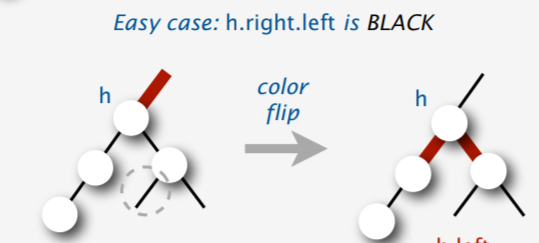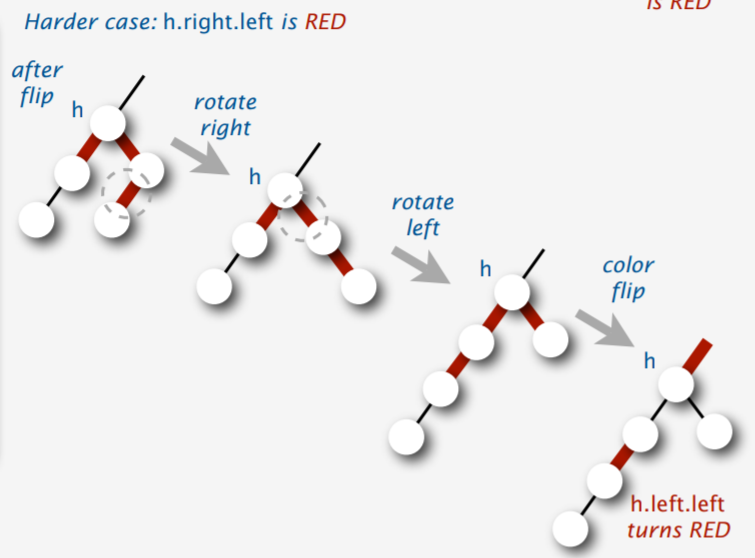template<class Key, class Compare>
typename Set<Key, Compare>::Node *
Set<Key, Compare>::move_red_left(Set::Node *root) const {
color_flip(root);
if (is_red(root->rc->lc)) {
// assume that root->rc != nullptr when calling this function
root->rc = rotate_right(root->rc);
root = rotate_left(root);
color_flip(root);
}
return root;
}

template<class Key, class Compare>
typename Set<Key, Compare>::Node *
Set<Key, Compare>::delete_min(Set::Node *root) const {
if (root->lc == nullptr) {
delete root;
return nullptr;
}
if (!is_red(root->lc) && !is_red(root->lc->lc)) {
// make sure either root->lc or root->lc->lc is red
// thus make sure we will delete a red node in the end
root = move_red_left(root);
}
root->lc = delete_min(root->lc);
return fix_up(root);
}



#### delete_arbitrary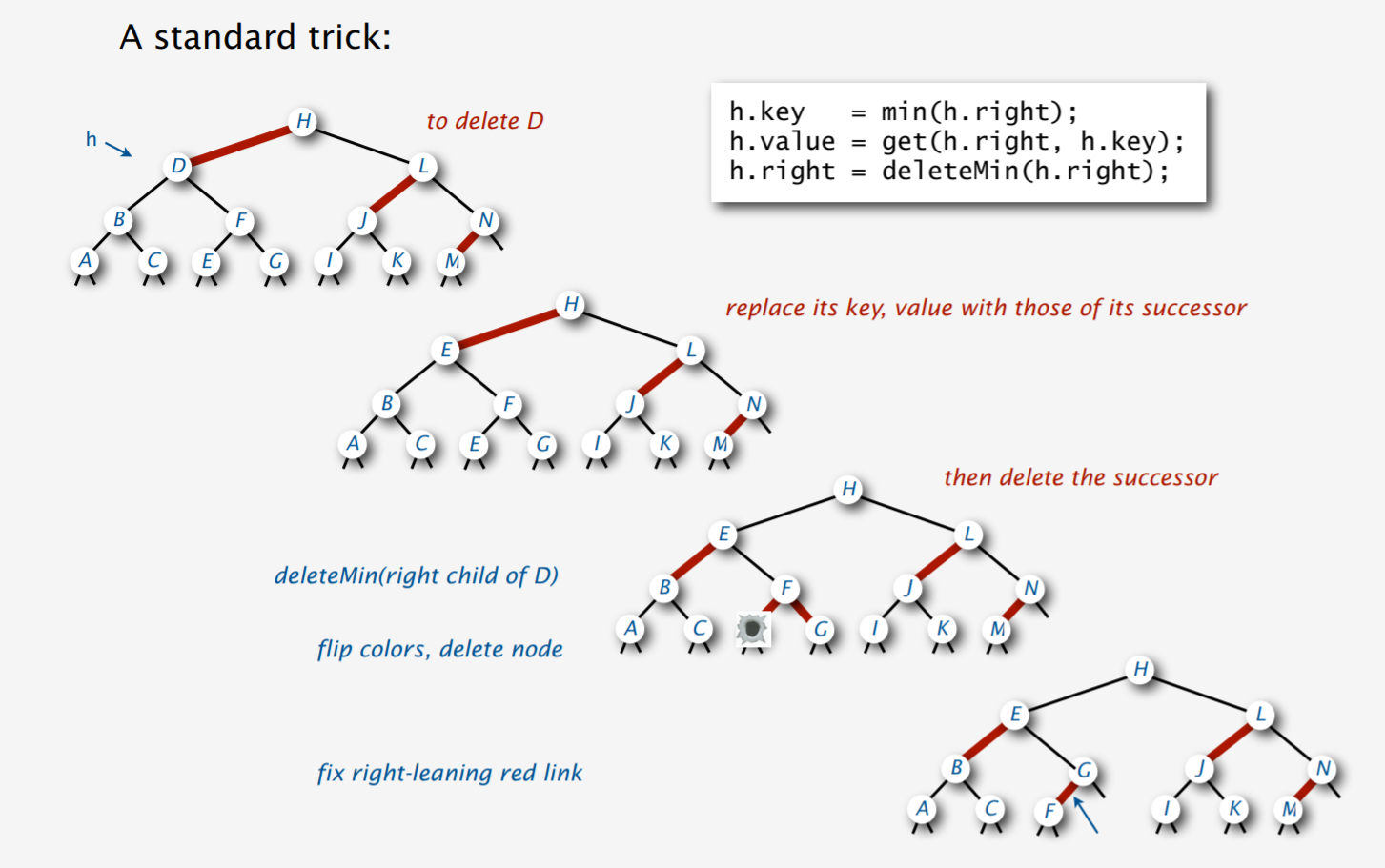template<class Key, class Compare>
typename Set<Key, Compare>::Node *
Set<Key, Compare>::delete_arbitrary(Set::Node *root, Key key) const {
if (cmp_(key, root->key)) {
// key < root->key
if (!is_red(root->lc) && !(is_red(root->lc->lc)))
root = move_red_left(root);
// ensure the invariant: either root->lc or root->lc->lc (or root and root->lc after dive into the function) is red,
// to ensure we will eventually delete a red node. therefore we will not break the black height balance
root->lc = delete_arbitrary(root->lc, key);
} else {
// key >= root->key
if (is_red(root->lc))
root = rotate_right(root);
if (key == root->key && root->rc == nullptr) {
delete root;
return nullptr;
}
if (!is_red(root->rc) && !is_red(root->rc->lc))
root = move_red_right(root);
if (key == root->key) {
root->key = get_min(root->rc);
root->rc = delete_min(root->rc);
} else {
root->rc = delete_arbitrary(root->rc, key);
}
}
return fix_up(root);

}